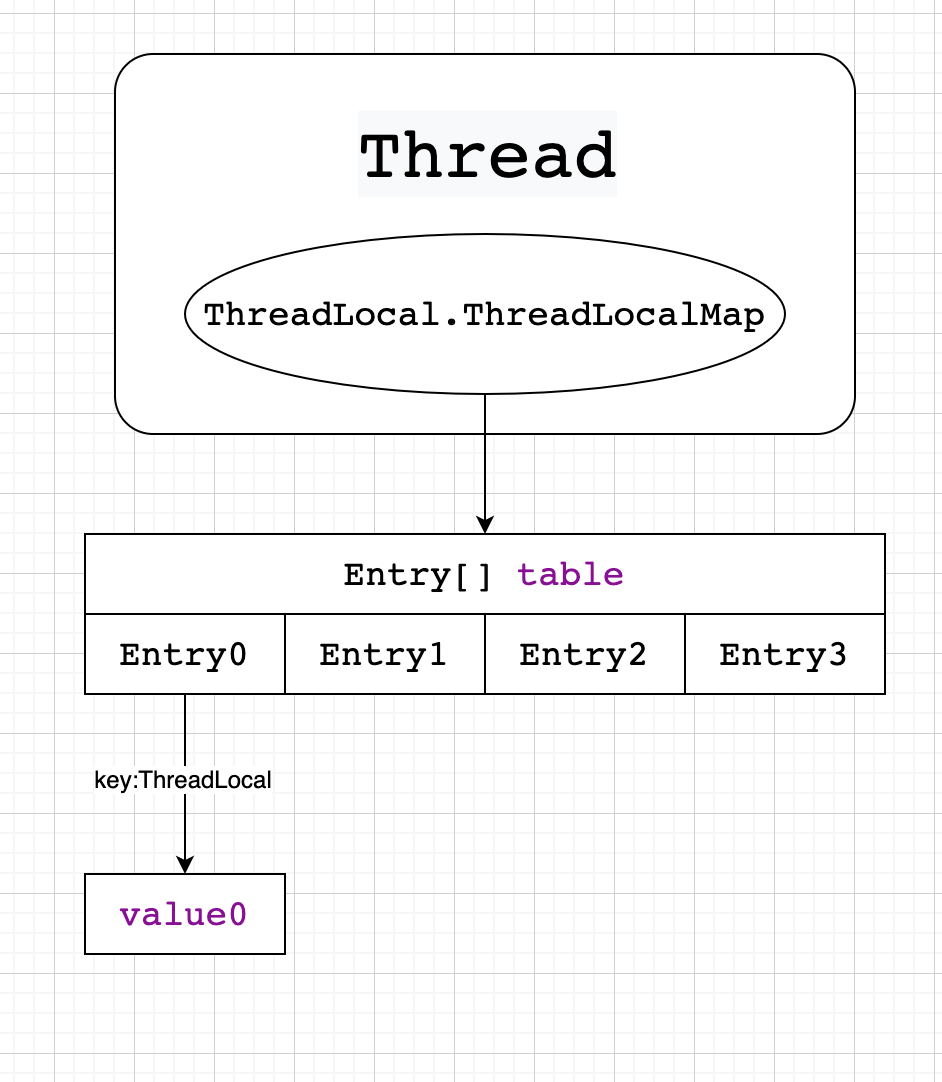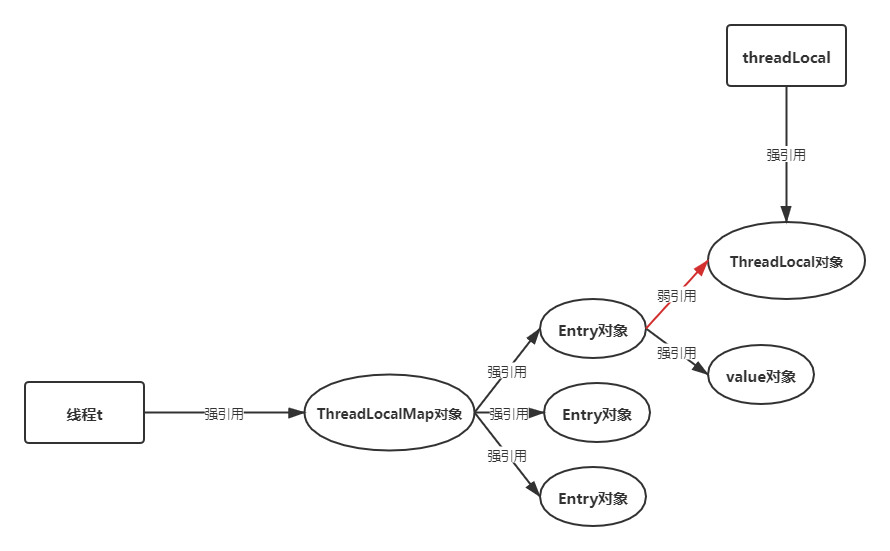``````public class UserHolder {

/**
* 存储用户信息
*/

/**
* 获取用户信息
*
* @return
*/
public User getUser() {
}

/**
* 设置用户信息
*
* @param user
*/
public void setUser(User user) {
}

/**
* 移除用户信息
*/
public void removeUser() {
}

}
``````

``````public class Test {

public static void main(String[] args) {
tl1.set(1);
tl1.get();
tl1.remove();
}

}
``````

2、从get、set方法入手

``````public T get() {
if (map != null) {
if (e != null) {
@SuppressWarnings("unchecked")
T result = (T)e.value;
return result;
}
}
return setInitialValue();
}

public void set(T value) {
if (map != null)
map.set(this, value);
else
createMap(t, value);
}
``````

3、基本模型``````ThreadLocalMap getMap(Thread t) {
}
``````

2、为什么Entry是一个WeakReference弱引用类型？

• 强引用：最常使用到的=赋值的情况就是强引用，对象只有在与GC Root之间没有强引用链的情况下才会被回收。
• 软引用：内存不够就回收，内存充足不回收。
• 弱引用：只要发生GC，一定被回收。
• 虚引用：是最弱的引用关系，无法通过虚引用来取得一个对象实例。为一个对象设置虚引用关联的唯一目的就是能在这个对象被收集器回收时收到一个系统通知。
``````static class Entry extends WeakReference<ThreadLocal<?>> {
/** The value associated with this ThreadLocal. */
Object value;

super(k);
value = v;
}
}
``````3、发生hash冲突时，也是使用链表或者红黑树吗？

``````private void set(ThreadLocal<?> key, Object value) {

// We don't use a fast path as with get() because it is at
// least as common to use set() to create new entries as
// it is to replace existing ones, in which case, a fast
// path would fail more often than not.

Entry[] tab = table;
int len = tab.length;
int i = key.threadLocalHashCode & (len-1);

for (Entry e = tab[i];
e != null;
e = tab[i = nextIndex(i, len)]) {

if (k == key) {
e.value = value;
return;
}

if (k == null) {
replaceStaleEntry(key, value, i);
return;
}
}

tab[i] = new Entry(key, value);
int sz = ++size;
if (!cleanSomeSlots(i, sz) && sz >= threshold)
rehash();
}
``````

``````private Entry getEntry(ThreadLocal<?> key) {
int i = key.threadLocalHashCode & (table.length - 1);
Entry e = table[i];
if (e != null && e.get() == key)
return e;
else
return getEntryAfterMiss(key, i, e);
}

/**
* Version of getEntry method for use when key is not found in
* its direct hash slot.
*
* @param  key the thread local object
* @param  i the table index for key's hash code
* @param  e the entry at table[i]
* @return the entry associated with key, or null if no such
*/
private Entry getEntryAfterMiss(ThreadLocal<?> key, int i, Entry e) {
Entry[] tab = table;
int len = tab.length;

while (e != null) {
if (k == key)
return e;
if (k == null)
expungeStaleEntry(i);
else
i = nextIndex(i, len);
e = tab[i];
}
return null;
}
``````

4、map是如何进行扩容的？

``````private void resize() {
Entry[] oldTab = table;
int oldLen = oldTab.length;
int newLen = oldLen * 2;
Entry[] newTab = new Entry[newLen];
int count = 0;

for (int j = 0; j < oldLen; ++j) {
Entry e = oldTab[j];
if (e != null) {
if (k == null) {
e.value = null; // Help the GC
} else {
int h = k.threadLocalHashCode & (newLen - 1);
while (newTab[h] != null)
h = nextIndex(h, newLen);
newTab[h] = e;
count++;
}
}
}

setThreshold(newLen);
size = count;
table = newTab;
}
``````